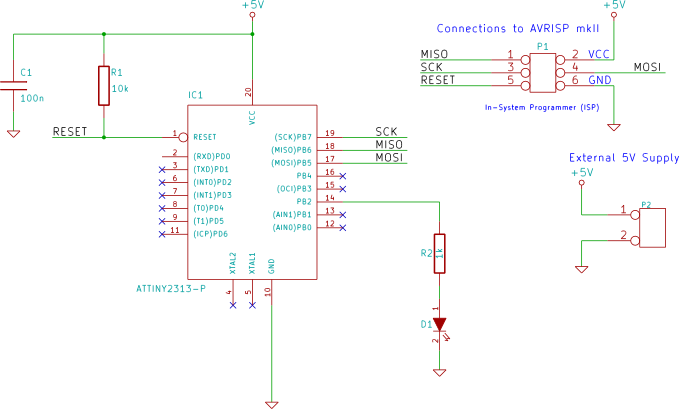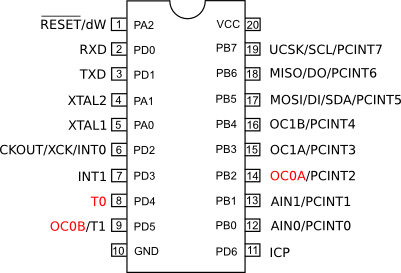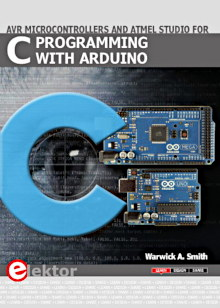Starting Electronics needs your help! Please make a donation to help cover our hosting and other costs. Click the donate button to send a donation of any amount.# ATtiny2313 PWM – Pulse Width Modulation

Created on: 6 May 2013

Part 11 of the ATtiny2313 Tutorial

Timer/Counter0 of the ATtiny2313 is used to generate a PWM (Pulse Width Modulation) waveform. PWM is demonstrated by using it to brighten and dim an LED.

This video shows the first example of PWM. The width of the pulse is changed every two seconds which brightens and dims the LED. The output from the PWM is also shown on an oscilloscope.

Can't see the video? View on YouTube →

## Circuit Diagram

The PWM output is obtained from the OC0A output of timer/counter0 which is on PB2, pin 14 of the ATtiny2313.Circuit Diagram Showing the LED Connected to PB2 which is the PWM Output OC0A from TC0ATtiny2313 with TC0 Pins Highlighted. OC0A is Used as the PWM Output

## Stepped PWM Example

This example changes the PWM value (and therefore the pulse width) every two seconds. This causes the LED to change to a different brightness every two seconds as shown in the video above.

### Software

```// TC0_PWM.c
#define F_CPU 1000000UL
#include <avr/io.h>
#include <util/delay.h>

int main(void)
{
DDRB   |= (1 << PB2);                   // PWM output on PB2
TCCR0A = (1 << COM0A1) | (1 << WGM00);  // phase correct PWM mode
OCR0A  = 0x10;                          // initial PWM pulse width

TCCR0B = (1 << CS01);   // clock source = CLK/8, start PWM

while(1)
{
// change PWM pulse width every 2 seconds
_delay_ms(2000);
OCR0A  = 0x10;
_delay_ms(2000);
OCR0A  = 0x30;
_delay_ms(2000);
OCR0A  = 0x50;
_delay_ms(2000);
OCR0A  = 0xA0;
}
}
```

Before using the PWM, the output pin from the PWM must be set as an output. This pin is PB2 which has the alternate function of output from Timer/Counter 0:

```DDRB   |= (1 << PB2);                   // PWM output on PB2
```

Timer/Counter0 is then put into phase correct PWM mode, set with an initial value and started.

Writing to the OCR0A will change the width of the PWM pulse.

### Project Source Code

The source code above can be copied and pasted to your own project, or download the Atmel Studio project for this example here:

TC0_PWM.zip (14.8kB)

Books that may interest you:## Continuous PWM Example

This example fades the LED from dim to bright and then back to dim continuously as shown in this video.

Can't see the video? View on YouTube →

### Software

```//TC0_PWM_fade.c
#define F_CPU 1000000UL
#include <avr/io.h>
#include <util/delay.h>

int main(void)
{
unsigned char PWM_val = 0;		// 8-bit PWM value
unsigned char up_dn = 0;		// up down count flag

DDRB   |= (1 << PB2);                   // PWM output on PB2
TCCR0A = (1 << COM0A1) | (1 << WGM00);  // phase correct PWM mode
TCCR0B = (1 << CS01);   // clock source = CLK/8, start PWM

while(1)
{
if ((PWM_val == 255) || (PWM_val == 0)) {
up_dn ^= 0x01;      // toggle count direction flag
}
_delay_ms(5);
OCR0A  = PWM_val;       // write new PWM value
if (up_dn) {            // increment or decrement PWM value
PWM_val++;
}
else {
PWM_val--;
}
}
}
```

By incrementing the value written to the OCR0A every 5ms, the brightness of the LED is increased smoothly. When the maximum value (255) that can be written to OCR0A is reached, the value is decremented every 5ms, smoothly dimming the LED.

The PWM in this example is set up in the same phase correct PWM mode as the previous example.

### Project Source Code

The source code above can be copied and pasted to your own project, or download the Atmel Studio project for this example here: